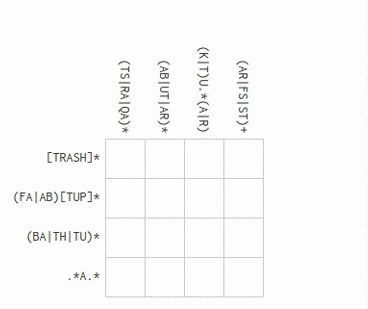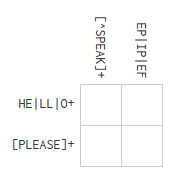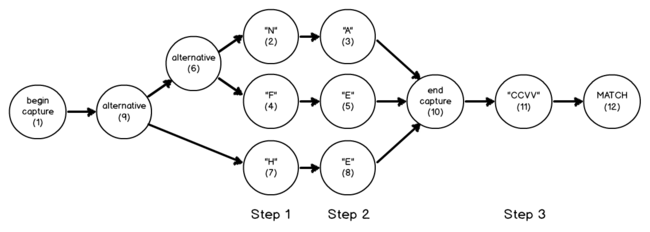I first discovered regular expression crosswords two weeks ago, when RegexCrossword.com appeared on the Hacker News front page. I thoroughly enjoyed the nerdy 30 minutes I spent doing the puzzles, but soon enough my natural inclination towards making computers do my hard work got the better of me: I wanted to solve this with code. This morning I had some free time and decided to give it a shot. It was perhaps even more fun, and definitely more exciting, than solving the puzzles by hand!

The end result is about 150 lines of Go code that solves most puzzles in only a few milliseconds. By showing you how I solved it, I hope you’ll learn some interesting things about solving puzzles programatically, how regular expressions work and specifically, how they work in Go. Let’s dive in.

### The algorithm

My first instinct was to solve this with brute force, by iterating over every possible answer to the puzzle, until a solution is found. This would work fine for small puzzles, like when the grid is 2x2. The solutions always use upper case characters, but they may also contain numbers and punctuation. So if we consider those 62 ASCII characters at every cell, the number of possible answers would be 62^4 for the 2x2 grid. At 14,776,336, that’s still a manageable search space for a brute force solution. But let’s make the grid 4x4, what then? Then we have 62^16, which is around 4.76x1028. Not so manageable anymore!

Then I thought about the strategy I use when solving these puzzles as a human. For every cell, I check what characters might satisfy the regular expressions for that cell. I then guess one of the options, and continue with the assumption that it’s correct. I do this until I find a contradiction, in which case I would go revisit my assumptions. It seems like this strategy of making assumptions and then backtracking when contradictions are found would work well as an algorithm, but there’s a snag: regular expression libraries don’t normally offer a way to tell whether the expression is matched only at a specific position. You either have to match the entire string, or nothing.

It would therefore be great if we had a function that could validate, for any given cell, whether a character could possibly satisfy the regular expression at that position. Let’s call this function satisfiesAtPos. It takes a regular expression expr, a character r and a position pos, and tells us whether the character is a possible match for this regular expression at position pos. For example, if we had this simple puzzle:then the first cell (in the left-hand upper corner) would be satisfied by H, L, or O based on the horizontal regex HE|LL|O+, so satisfiesAtPos should return true for these characters. Anything that’s not in the set {S,P,E,A,K} would satisfy vertical regex [^SPEAK] at position 0, so satisfiesAtPos would return false for {S,P,E,A,K}, and true for all other characters. This means only H will have satisfiesAtPos return true for both regexes at position 0. We can continue in this fashion for every cell, until we reach the end of a row or column, where we should then validate our assumptions for the independent cells. If the row or column doesn’t match the regular expression, we backtrack and try other options for the cells where there is more than one possibility.

### Regular Expressions and Go

We have an algorithm! Now we just need to figure out how to write the function satisfiesAtPos. In Go, it’s frighteningly easy if you understand some regular expression theory and have the patience to scratch around in the source code. For understanding regular expression theory, this article by Russ Cox is an excellent introduction on the subject. And since the author is also one of the core contributors to Go, the algorithm described in this article is also the one implemented in the Go regexp package. Convenient!

I’m not going to re-iterate everything said in that article, and I highly recommend reading it. But as long as you know that regular expressions can be expressed as state machines, you should be able to follow the rest of this discussion.

Normally when you use regular expressions in Go, you call the regexp package. Specifically, you might call regexp.Compile, like this:

re, err := regexp.Compile(HE|LL|O+)


which gives you re, a *Regexp you can use to call Match, Find, or Replace. But what does Go do internally to get you that Regexp struct? It’s very interesting.

When you call regexp.Compile, a number of things happen: - first, syntax.Parse gets called. This parses a regular expression string s, controlled by the specified flags, and returns a regular expression parse tree. Next, - the regular expression gets simplified by calling Simplify, which returns a regexp equivalent to the given one but without counted repetitions and with various other simplifications, such as rewriting /(?:a+)+/ to /a+/. And last, - syntax.Compile compiles the regexp into a program that can be executed. This step returns a syntax.Prog, which is a state machine, and it’s what gets used to run match checks. It has a String() method that spits out its internals in human-readable format, and this is really useful in understanding regular expressions in Go.

Here’s a simple program that performs the steps described above, and prints out the resulting program (state machine):

package main

import (
"fmt"
"regexp/syntax"
)

var flags = syntax.MatchNL | syntax.PerlX | syntax.UnicodeGroups

func main() {
re, err := syntax.Parse("(NA|FE|HE)[CV]", flags)
if err != nil {
panic(err)
}

re = re.Simplify()
prog, err := syntax.Compile(re)
if err != nil {
panic(err)
}
fmt.Println(prog)
}


Running this gives us the following output:

  0	fail
1*	cap 2 -> 9
2	rune1 "N" -> 3
3	rune1 "A" -> 10
4	rune1 "F" -> 5
5	rune1 "E" -> 10
6	alt -> 2, 4
7	rune1 "H" -> 8
8	rune1 "E" -> 10
9	alt -> 6, 7
10	cap 3 -> 11
11	rune "CCVV" -> 12
12	match


This may seem cryptic at first, but it’s actually not hard to understand. The output describes a state machine. The first number on each line is the ID of the node. The next word describes the type of node. It could be cap, which is short for capture, and corresponds to the start and end of match groups in the regex. rune1 describes a node that exactly matches a specific rune. alt describes a node that merely exists to create a fork in the road: one path leads to the first argument, and the other to the second argument. And so on for different types of nodes. Every node has an output, described by the final number, which tells us the ID of the next node after this one. All in all, the above gives us a state machine diagram that looks more or less like this:You’ll notice that I annotated the above state diagram with three steps. What are they? They are what we need to solve our initial problem: determinining whether a given character can be a solution to the regex at a particular step. If we want to know whether 'N' satisfies the regular expression at position zero, we check whether 'N' is a possible match at step one. If it is, satisfiesAtPos should return true, otherwise false. The problem is almost solved.

I conveniently structured the state machine diagram so that rune matches fall on the same step for every position in the string. How do we do this in code? We can build up a list of runes at a particular step by stepping through the state machine, starting at the starting node. We start with a queue containing only the starting node, and we iterate until the queue is empty. If the next node in the queue is a rune node, we add it to our list of nodes at this step. If the node is not a rune, but rather an alt (alternative, or fork in the road) or cap (capture), we add this node’s output nodes to the queue. Ideally, the queue should be a set, so that we can’t add the same node to it twice. And that’s how it’s done; we can now complete our satisfiesAtPos function. In Go code, it looks like this:

func satisfiesAtPos(expr string, r rune, pos int) bool {
re, err := syntax.Parse(expr, flags)
if err != nil {
panic(err)
}

re = re.Simplify()
prog, err := syntax.Compile(re)
if err != nil {
panic(err)
}

var el int
s := 0                          // current step
step := []int{}                 // states in this step
queue := set([]int{prog.Start}) // queue of states to check

for s <= pos {
for len(queue) > 0 {
el, queue = queue, queue[1:]
inst := prog.Inst[el]
switch inst.Op {
case syntax.InstAlt:
// alternative, so add both nodes to queue
case syntax.InstCapture:
// capture group, just add next node to queue
case syntax.InstRune, syntax.InstRune1, syntax.InstRuneAny:
// rune, add instance to step
step = append(step, el)
}
}

// range over the nodes we found in this step
for i := range step {
inst := prog.Inst[step[i]]
if s == pos && inst.MatchRune(r) {
// if this is the step we want and
// the rune matches, return true
return true
}
// add rune's out to queue
queue = append(queue, int(inst.Out))
}
step = []int{}
s++
}

return false
}


### Putting it all together

Now that we have a working satisfiesAtPos function, the rest is relatively easy. For every cell, we guess a character and call satisfiesAtPos for both the horizontal and vertical regular expression. If the character satisfies both regexes, we go on to the next cell. If not, we guess the next character, and repeat the process. At the end of a row, we validate the row’s regular expression, and if it doesn’t match, we backtrack and keep making different guesses until it does. We also do the same for the columns. Even though we are still iterating over possible answers, we quickly find out when an answer cannot lead to a correct solution, and stop the search in its tracks. This reduces the search space considerably.

If you are interested in the code for this function, feel free to check out the Github repo.

### Caveats

If you read the article by Russ Cox I recommended earlier, you might have noticed the section on backreferences. Specifically, how they cannot be implemented in the way described in the article. This is why Go does not support regular expressions with backreferences at the moment. A “particularly clever” solution might one day combine the two algorithms so that the fast Thompson NFA is used when backreferences are not present, and only bring out backtracking when needed. Unfortunately, this also means that my current solution for regex crosswords, though simple, short and reasonably efficient, does not support backreferences. I’m still thinking of ways to remedy this without making the code horribly complicated. Let me know if you have any ideas!

We also technically don’t need to build the program every time inside the satisfiesAtPos function. A simple, but effective, optimization would be to build the program only once, and then compile the steps only once. This will surely make the solution much faster, but so far, for all the puzzles I tried, solutions were found in reasonable time, mostly within a few milliseconds.

I have also not yet gotten around to adding support for puzzles with regular expressions on all four sides. I see no reason for it to be difficult, though.

### Grab the code

The code is available on Github. It is not hard to hook up Selenium (or something similar), but let me not encourage such anti-social behavior :) Have fun!